# Closed geodesic

(diff) ← Older revision | Latest revision (diff) | Newer revision → (diff)

A closed smooth curve on a Riemannian manifoldthat is a geodesic line. A more general notion is that of a geodesic loop, i.e. a geodesic(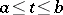) passing through the same pointat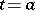and; considered as a closed curve, it may have an angle at. A geodesic loop is a closed geodesic only if it has no angle, i.e. if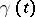has the same tangent atand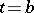. Closed trajectories of the geodesic flow in the tangent bundle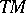ofare projected onto closed geodesics under the natural projection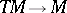. The curve obtained when the same closed geodesic is traversed several times is called a multiple closed geodesic. A closed geodesic that is not multiple is called a simple closed geodesic.

The definition of a closed geodesic and a geodesic loop carries over word for word to the case in whichis equipped with a Finsler metric or an affine connection. Ifis a metric space (in that case geodesic lines are defined as locally shortest lines), the definition of a geodesic loop remains the same, but that of a closed geodesic must be slightly modified since the notion of smoothness or angle are non-existent. A geodesic loop(), withandnot constant on any subinterval, is a closed geodesic if, for sufficiently small, the line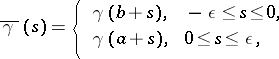i.e. the union of two arcs of: the first joining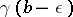to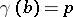, the second joiningto, is the shortest line between its end pointsand.

Closed geodesics have been investigated mainly in the case of closed Riemannian manifolds; there are also various results for Finsler manifolds; some results have been obtained in the more general case of metric spaces with certain special properties (known as Busemann-spaces; see Geodesic geometry) . These studies were initiated by J. Hadamard , H. Poincaré  and G. Birkhoff .

Hadamard was the pioneer in research on (not necessarily closed) geodesic lines on manifolds of negative curvature. The principal modern results relate to the case of closed manifoldsthe curvature of which is negative in all two-dimensional directions at all points. For closed geodesics on such manifoldsit has been proved that the closed trajectories of a geodesic flow are everywhere dense in(see ) (an analogous phenomenon has been observed in several examples with positive curvature, though not always ); there exists an estimate for the growth of the number of closed geodesics of length not exceeding, as a function of increasing(see ). Hadamard approximated long segments of non-closed geodesics by closed geodesics — this can be regarded as the beginning of symbolic dynamics.

Another theorem due to Hadamard provides some information on closed geodesics on a closed manifold(with no assumptions concerning its curvature) in terms of the properties of the fundamental group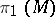. An oriented closed curvedefines a certain conjugacy class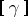in; all curves in the same conjugacy class may be derived from one another by a free homotopy of curves. It turns out that, for every conjugacy classof, except the conjugacy class corresponding to the identity element, the set of closed curveswith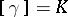contains shortest members, and these shortest curves are closed geodesics. This theorem (which is valid not only for Riemannian or Finsler metrics, but also in the general case of Busemann-spaces) is the simplest and historically the first result of the variational calculus in the large. However, the latter arose as an independent field of research from a more sophisticated application of variational methods to the study of closed geodesics on manifolds homeomorphic to a sphere, for which (as, in general, for simply-connected manifolds) the above theorem is meaningless.

The study of closed geodesics on such manifolds was proposed by Poincaré (to be precise: He was concerned with an ovaloid, i.e. a two-dimensional closed convex surface). In the light of the discussion in , the following conjecture arose: In the "general" case, there exist three closed geodesics without self-intersections on an ovaloid; two of them are stable in the linear approximation, while the other is unstable. This connection between the existence of closed geodesics and the estimation of their number with the question of their stability properties constitutes an essential feature of Poincaré's heuristic arguments, which mainly were related to the theory of dynamical systems. These arguments may be carried out on a perfectly rigorous level, but only for metrics which are sufficiently close to the "standard" metric (the ordinary metric on the spherein Euclidean space; see ). For metrics far away from the standard metric, the stability properties postulated by Poincaré for closed geodesics need not be valid .

The credit for the initiation of the variational approach to closed geodesics on simply-connected manifolds is due to Birkhoff . He proved that there is at least one closed geodesic on a manifold homeomorphic to a sphere. Later, Poincaré's conjecture concerning the existence of three non-self-intersecting closed geodesics on the two-dimensional sphere was proved by L.A. Lyusternik and L.G. Shnirel'man (see ).

Research has been done on the properties of closed geodesics for "typical" Riemannian (or Finsler) metrics (i.e. metrics forming sets of the second category in the space of all metrics of a given smoothness class) (see , ).

The standard metric on the sphere possesses the property that all its geodesics are closed and of the same length; there are also other metrics on the sphere with this property . Consideration has also been given to the question of the topological properties of manifolds and their metrics when the latter have the above-mentioned property or some variant thereof.

A detailed exposition of the theory of closed geodesics may be found in .

How to Cite This Entry:
Closed geodesic. Encyclopedia of Mathematics. URL: http://encyclopediaofmath.org/index.php?title=Closed_geodesic&oldid=19129
This article was adapted from an original article by D.V. Anosov (originator), which appeared in Encyclopedia of Mathematics - ISBN 1402006098. See original article